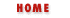#Chapter 4Expressions and Operators## 4.3 Arithmetic Operators

Having explained operator precedence, associativity, and other background material, we can start to describe the operators themselves. This section details the arithmetic operators.

The + operator adds its two numeric operands. If both operands are strings, then it returns a string that is the result of concatenating the second operand onto the first. If either operand is a string, then the other is converted to a string, and the two strings are concatenated. Furthermore, if either operand is an object, then both operands are converted to strings and concatenated.

### Subtraction (-)

The - operator subtracts its second operand from its first. Both operands must be numbers. Used as a unary operator, - negates its operand.

### Multiplication (*)

The * operator multiplies its two operands, which must both be numbers.

### Division (/)

The / operator divides its first operand by its second. Both operands must be numbers. If you are a C programmer, you might expect to get an integer result when you divide one integer by another. In JavaScript, however, all numbers are floating-point, so all divisions have floating-point results: 5/2 evaluates to 2.5, not 2.

### Modulo (%)

The % operator computes the first operand modulo the second operand. That is, it returns the remainder when the first operand is divided by the second operand an integer number of times. Both operands must be numbers. For example, 5 % 2 evaluates to 1.

While the modulo operator is typically used with integer operands, it also works for floating-point values. For example, 4.3 % 2.1 == 0.1.

### Unary Negation (-)

When - is used as a unary operator, before a single operand, it performs unary negation, i.e., it converts a positive value to an equivalently negative value, and vice versa.

### Increment (++)

The ++ operator increments (i.e., adds 1 to) its single operand, which must be a variable, an element of an array, or a property of an object that refers to a numeric value. The precise behavior of this operator depends on its position relative to the operand. When used before the operand, where it is known as the pre-increment operator, it increments the operand and evaluates to the incremented value of that operand. When used after the operand, where it is known as the post-increment operator, it increments its operand, but evaluates to the unincremented value of that operand.

For example, the following code sets both i and j to 2:

```i = 1;
j = ++i;
```
But these lines set i to 2 and j to 1:

```i = 1;
j = i++;
```
This operator, in both its forms, is most commonly used to increment a counter that controls a loop.

### Decrement (--)

The -- operator decrements (i.e., subtracts 1 from) its single numeric operand, which must be a variable, an element of an array, or a property of an object. Like the ++ operator, the precise behavior of -- depends on its position relative to the operand. When used before the operand, it decrements and returns the decremented value. When used after the operand, it decrements, but returns the undecremented value.Operator OverviewComparison Operators